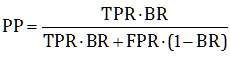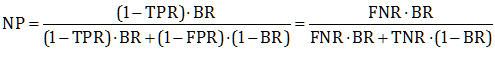This is a compendium of definitions used throughout the blog:

H = Investigated Hypothesis

E = Evidence about H. Can be positive (present) or negative (absent)

P(H) = BR = Base Rate. Unconditional, prior probability. Probability of H before the arrival of E

P(E|H) = TPR = True Positive Rate: Probability of positive E, given that H is true

P(E|not H) = FPR = False Positive Rate: Probability of positive E, given that H is false

P(not E|not H) = TNR = 1–FPR = True Negative Rate: Probability of negative E, given that H is false

P(not E|H) = FNR = 1–TPR = False Negative Rate: Probability of negative E, given that H is true

P(H|E) = PP = Probability that H is true, given positive E

P(H|not E)= NP = Probability that H is true, given negative E

Bayes’ Theorem:A = (TPR+TNR)/2 = 0.5 + (TPR–FPR)/2 = 0.5 + (TNR–FNR)/2 = Accuracy

BO = BR/(1–BR) = Prior (Base) Odds of H

PO = PP/(1–PP) = Posterior Odds of H, given positive E

NO = NP/(1–NP) = Posterior Odds of H, given negative E

LR = TPR/FPR = Likelihood Ratio of positive E

LRN = FNR/TNR = Likelihood Ratio of negative E

Bayes’ Theorem in Odds form:

PO = LR ∙ BO

NO = LRN ∙ BO